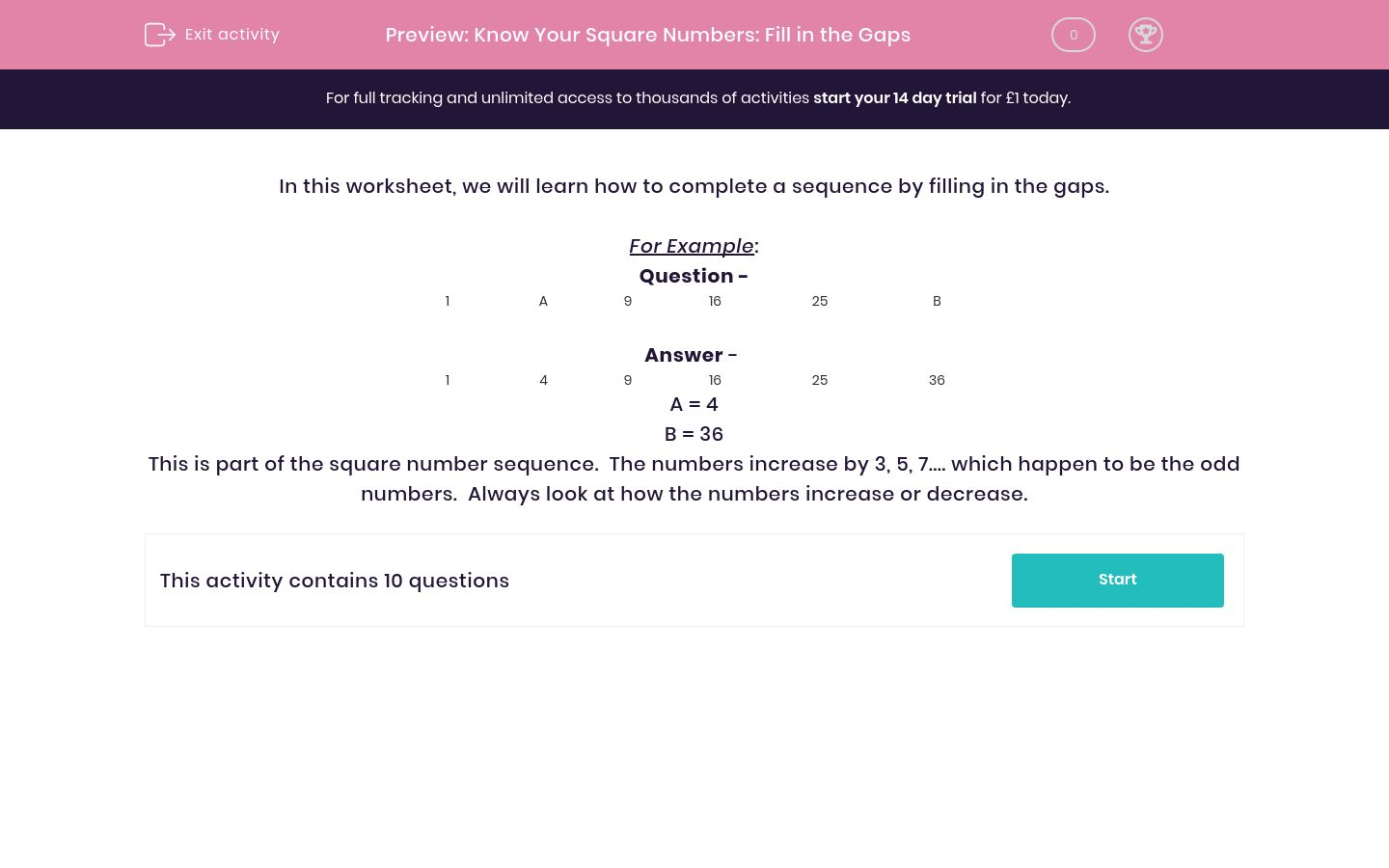# Know Your Square Numbers: Fill in the Gaps

In this worksheet, students complete number sequences by looking at the gaps between numbers.Key stage:  KS 2

Curriculum topic:   Maths and Numerical Reasoning

Curriculum subtopic:   Place Value

Difficulty level:### QUESTION 1 of 10

In this worksheet, we will learn how to complete a sequence by filling in the gaps.

For Example:

Question -

 1 A 9 16 25 B

 1 4 9 16 25 36

A = 4

B = 36

This is part of the square number sequence.  The numbers increase by 3, 5, 7.... which happen to be the odd numbers.  Always look at how the numbers increase or decrease.

Complete the sequence by filling in the gaps:

 1 3 6 A B 21
 Missing Numbers A B

Complete the sequence by filling in the gaps:

 42 A B C 82 92
 Missing Numbers A B C

Complete the sequence by filling in the gaps:

 16 A 34 43 B C
 Missing Numbers A B C

Complete the sequence by filling in the gaps:

 A 30 B 56 C 82
 Missing Numbers A B C

Complete the sequence by filling in the gaps:

 A B 77 91 105 C
 Missing Numbers A B C

Complete the sequence by filling in the gaps:

 33 20 A B C -32
 Missing Numbers A B C

Complete the sequence by filling in the gaps:

 A 36 B 10 -3 C
 Missing Numbers A B C

Complete the sequence by filling in the gaps:

 A B C 20 5 -10
 Missing Numbers A B C

Complete the sequence by filling in the gaps:

 A B 13 2 C -20
 Missing Numbers A B C

Complete the sequence by filling in the gaps:

 66 54 A B C 6
 Missing Numbers A B C
• Question 1

Complete the sequence by filling in the gaps:

 1 3 6 A B 21
 Missing Numbers A B
EDDIE SAYS
These are the triangle numbers. The numbers increase by 2, 3, 4, 5 etc....
• Question 2

Complete the sequence by filling in the gaps:

 42 A B C 82 92
 Missing Numbers A B C
EDDIE SAYS
The numbers increase by 10 each time.
• Question 3

Complete the sequence by filling in the gaps:

 16 A 34 43 B C
 Missing Numbers A B C
EDDIE SAYS
The numbers increase by 9 each time.
• Question 4

Complete the sequence by filling in the gaps:

 A 30 B 56 C 82
 Missing Numbers A B C
EDDIE SAYS
The numbers increase by 13 each time.
• Question 5

Complete the sequence by filling in the gaps:

 A B 77 91 105 C
 Missing Numbers A B C
EDDIE SAYS
The numbers increase by 14 each time.
• Question 6

Complete the sequence by filling in the gaps:

 33 20 A B C -32
 Missing Numbers A B C
EDDIE SAYS
The numbers decrease by 13 each time.
• Question 7

Complete the sequence by filling in the gaps:

 A 36 B 10 -3 C
 Missing Numbers A B C
EDDIE SAYS
The numbers decrease by 13 each time.
• Question 8

Complete the sequence by filling in the gaps:

 A B C 20 5 -10
 Missing Numbers A B C
EDDIE SAYS
The numbers decrease by 15 each time.
• Question 9

Complete the sequence by filling in the gaps:

 A B 13 2 C -20
 Missing Numbers A B C
EDDIE SAYS
The numbers decrease by 11 each time.
• Question 10

Complete the sequence by filling in the gaps:

 66 54 A B C 6
 Missing Numbers A B C
EDDIE SAYS
The numbers decrease by 12 each time.
---- OR ----

Sign up for a £1 trial so you can track and measure your child's progress on this activity.

### What is EdPlace?

We're your National Curriculum aligned online education content provider helping each child succeed in English, maths and science from year 1 to GCSE. With an EdPlace account you’ll be able to track and measure progress, helping each child achieve their best. We build confidence and attainment by personalising each child’s learning at a level that suits them.

Get started